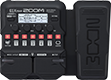# Base Clean com modução

Discussion in 'Zoom G1/G1X Four' started by acalegione, Mar 22, 2021.

1. Base Clean com moduçãoDevice: Zoom G1X Four
Firmware: 2.00

Name on device: BaseClnMod
Optimized for: Guitar Amp

Effects chain:Path para base, com leve modulação e reverb

Effect: "ZNR" (Dynamics), active - "yes"
"Detect" = EFXIN
"Depth" = 48
"Threshold" = 54
"Decay" = 5

Effect: "Aco.Sim" (Overdrive / Distortion), active - "yes"
"Top" = 80
"Body" = 50
"Tone" = 52
"Volume" = 100

Effect: "Gt GEQ 7" (Filter), active - "yes"
"100" = 0.5
"200" = -6.0
"400" = 5.5
"800" = 0.0
"1.6k" = -4.0
"3.2k" = -3.5
"6.4k" = 8.0
"VOL" = 80

Effect: "StereoCho" (Modulation), active - "yes"
"Depth" = 85
"Rate" = 30
"Tone" = 6
"Mix" = 50

Effect: "HD Hall" (Reverb), active - "yes"
"PreD" = 81
"Decay" = 45
"Mix" = 62
"Tail" = Off

Patch Volume: 97